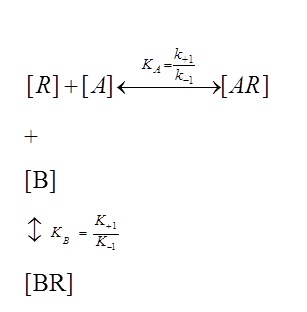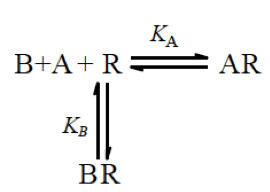Gaddum modification of Operational Model of Efficacy

The effect of an antagonist on the efficacy of an agonist is based on the following equilibrium relationship wherein the empty receptor (R) competes with the antagonist (B) and the receptor can exist in an antagonist bound state (BR) as well:When applying the law of mass action to these simultaneous equations, we can derive the following relationship: Continue reading

Del Castillo-Katz model of Efficacy

The standard model of measuring efficacy as a function of receptor occupancy was deemed to be insufficient to adequately explain the dose-response relationships often observed, that were steeper than those predicted by the law of mass action; or why sometimes a supra maximal drug concentration failed to elicit a maximal response within previously observed systems. This was predominantly manifested by a lack of a linear correlation, as predicted by the hyperbolic Langmuir isotherm, between the observed responses of a ligand on its tissues versus the fractions of occupied receptors. In 1956, R. P. Stephenson argued that the magnitude of response, in a tissue, to a given stimulus was the product of the intrinsic efficacy$(e_A)$ of the agonist and the fractions of occupied receptors,$\rho_{AR}$, as defined by the Hill-Langmuir equation: Continue reading

What happens to the log (concentration) vs response curve of a drug in the presence of a competitive antagonist? Assuming that the agonist and the antagonist are in equilibrium with the receptor-binding site, we will observe a parallel shift in the agonist concentration response curve. We can estimate the proportion of agonist and antagonist bound to the receptors by the concentration of the agonist and antagonist and by their affinities for the receptor as follows:Under the laws of Mass Action, we can derive the following relationship: Continue reading

Derivation of Hill Langmuir Equation

A simple reaction scheme for the interaction of an agonist [A] with its receptor [R] can be described as:Rate of forward reaction:$k_{+1}$

Rate of reverse reaction:$k_{-1}$

Black and Leff Operational model of Efficacy

This modified definition of receptor occupancy defined by the del Castillo-Katz model now allows us to re-define R. P. Stephenson’s original postulate in Eq. (1.0) as follows:$Stimulus (SA) = e^*_A \delta_{occupied(A)} = S = e^*_A \frac {[A]}{K_{effective} + [A]}$                                     (1.29)